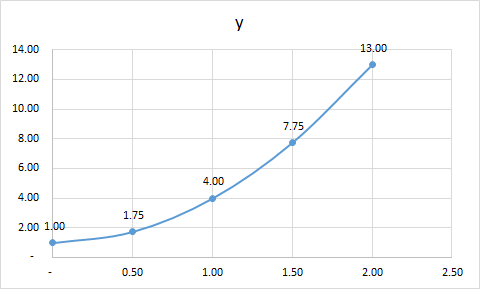# Use the definition of the definite integral to evaluate ∫02 (3x2 +1)dx. Use a right-endpoint approximation to generate the Riemann sum.

Question
53 views

Use the definition of the definite integral to evaluate ∫02 (3x2 +1)dx. Use a right-endpoint approximation to generate the Riemann sum.

check_circle

Step 1

As a first step, let's plot the graph of the curve given to us. Let's call the curve y = f(x) = (3x2 +1).

Please see the plot of y vs x in the range x = 0 to x = 2 at an interval of 0.50Step 2

The rectangles using right point have been put on the graph. We have to now calculate the area of the rectangles and sum them. Please see the white board.

Area of a rectangle = Length x width

Width of each rectan...

### Want to see the full answer?

See Solution

#### Want to see this answer and more?

Solutions are written by subject experts who are available 24/7. Questions are typically answered within 1 hour.*

See Solution
*Response times may vary by subject and question.
Tagged in

### Calculus